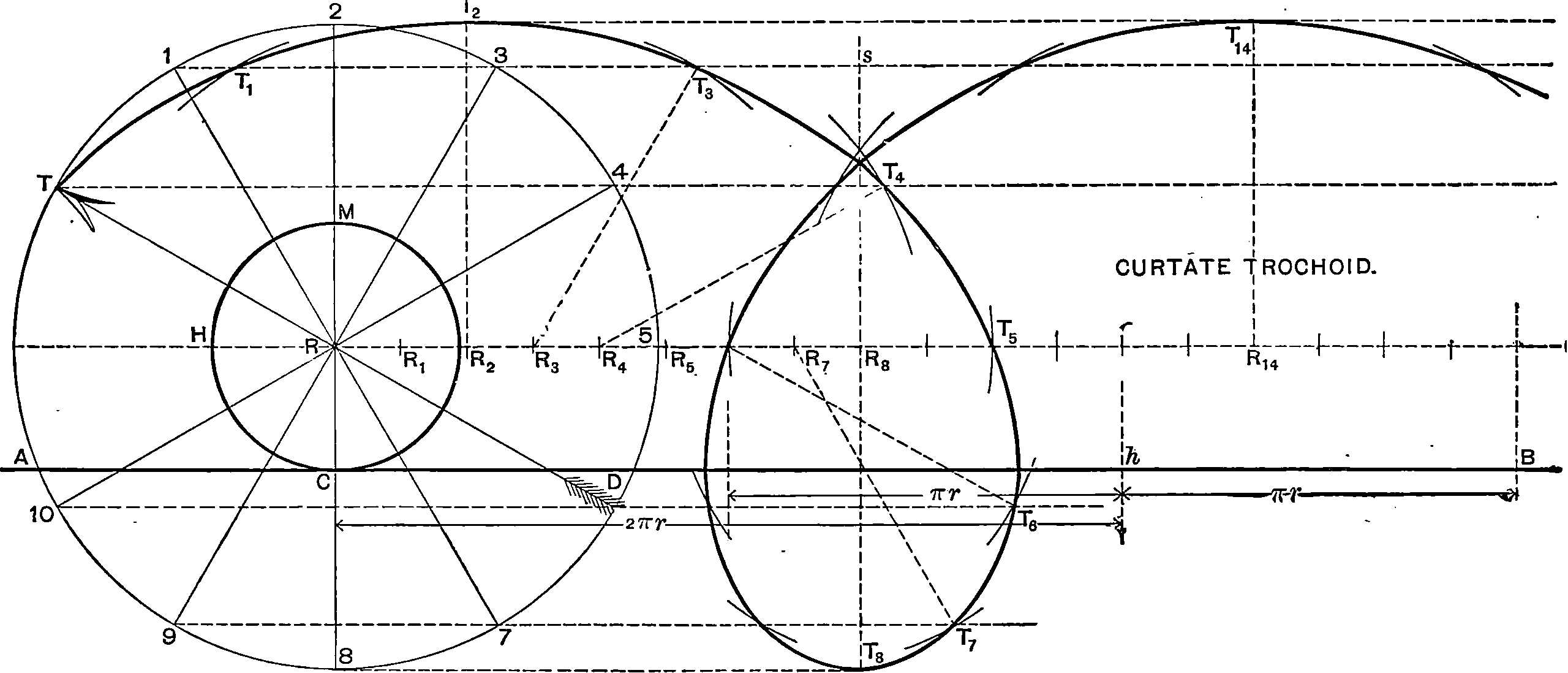# Recursive identification

## Learning forward dynamics by looking at time series.

A grab-bag of perspectives and tricks for recursive identification of dynamical systems, i.e. updating a model which produces the correct forward predictions, given the past.Keywords: multi-step prediction, time horizon, teacher forcing. The various things that are meant by “autoregressive”.

A common core of ideas pop up here in forecasting and state filtering system identification (including particle version), RNNs and forward operator learning. We could describe Koopman operator as an alternative perspective.

## Classic systems learning

Landmark papers according to Lindström et al. (2012):

Augmenting the unobserved state vector is a well known technique, used in the system identification community for decades, see e.g. Ljung . Similar ideas, using Sequential Monte Carlos methods, were suggested by . Combined state and parameter estimation is also the standard technique for data assimilation in high-dimensional systems, see Moradkhani et al.

However, introducing random walk dynamics to the parameters with fixed variance leads to a new dynamical stochastic system with properties that may be different from the properties of the original system. That implies that the variance of the random walk should be decreased, when the method is used for offline parameter estimation, cf. .

## The pushforward trickWhen writing Takamoto et al. (2022) we learned a useful way of thinking about this problem from Brandstetter, Worrall, and Welling (2022), which solved many difficulties at once for us. They think about it as a distribution shift problem, but one where we can reduce the magnitude of the implied distribution shift, which they call the pushforward trick.

We approach the problem in probabilistic terms. The solver maps $$p_k \mapsto$$ $$\mathcal{A}_{\sharp} p_k$$ at iteration $$k+1$$, where $$\mathcal{A}_{\sharp}: \mathbb{P}(X) \rightarrow \mathbb{P}(X)$$ is the pushforward operator for $$\mathcal{A}$$ and $$\mathbb{P}(X)$$ is the space of distributions on $$X$$. After a single test time iteration, the solver sees samples from $$\mathcal{A}_{\sharp} p_k$$ instead of the distribution $$p_{k+1}$$, and unfortunately $$\mathcal{A}_{\sharp} p_k \neq p_{k+1}$$ because errors always survive training. The test time distribution is thus shifted, which we refer to as the distribution shift problem. This is a domain adaptation problem. We mitigate the distribution shift problem by adding a stability loss term, accounting for the distribution shift. A natural candidate is an adversarial-style loss $L_{\text {stability }}=\mathbb{E}_k \mathbb{E}_{\mathbf{u}^{k+1} \mid \mathbf{u}^k, \mathbf{u}^k \sim p_k}\left[\mathbb{E}_{\boldsymbol{\epsilon} \mid \mathbf{u}^k}\left[\mathcal{L}\left(\mathcal{A}\left(\mathbf{u}^k+\boldsymbol{\epsilon}\right), \mathbf{u}^{k+1}\right)\right]\right]$ where $$\epsilon \mid \mathbf{u}^k$$ is an adversarial perturbation sampled from an appropriate distribution. For the perturbation distribution, we choose $$\epsilon$$ such that $$\left(\mathbf{u}^k+\boldsymbol{\epsilon}\right) \sim \mathcal{A}_{\sharp} p_k$$. This can be easily achieved by using $$\left(\mathbf{u}^k+\boldsymbol{\epsilon}\right)=\mathcal{A}\left(\mathbf{u}^{k-1}\right)$$ for $$\mathbf{u}^{k-1}$$ one step causally preceding $$\mathbf{u}^k$$. Our total loss is then $$L_{\text {one-step }}+L_{\text {stability. }}$$ We call this the pushforward trick. We implement this by unrolling the solver for 2 steps but only backpropagating errors on the last unroll step, as shown in Figure. … This is not only faster, it also seems to be more stable. Exactly why, we are not sure, but we think it may be to ensure the perturbations are large enough. Training the adversarial distribution itself to minimize the error, defeats the purpose of using it as an adversarial distribution. Adversarial losses were also introduced in Sanchez-Gonzalez et al. (2020) and later used in Mayr et al. (2023), where Brownian motion noise is used for $$\epsilon$$ and there is some similarity to Noisy Nodes , where noise injection is found to stabilize training of deep graph neural networks. There are also connections with zero-stability (Hairer et all, 1993) from the ODE solver literature. Zero-stability is the condition that perturbations in the input conditions are damped out sublinearly in time, that is $$\left\|\mathcal{A}\left(\mathbf{u}^0+\epsilon\right)-\mathbf{u}^1\right\|<\kappa\|\boldsymbol{\epsilon}\|$$, for appropriate norm and small $$\kappa$$. The pushforward trick can be seen to minimize $$\kappa$$ directly.

That is an interesting justification for a very simple trick; we train better by using a two steps forward-one step back approach, where the forward step is a pushforward of the previous step.Brandstetter, Worrall, and Welling (2022)’s answer to the distribution shift problem.

## Backpropagation through time

How we discuss learning parameters with classic recurrent neural networks .

We can think of the problem of learning recurrent networks as essentially a system identification problem with all the implied difficulties including stability problems.

RNN research has its own special terminology, e.g. vanishing/exploding gradients . TBPTT (truncated back propagation through time), which makes explicit with respect to when gradients are taken.